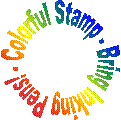Mathman 31 A Playground Puzzle Letterbox by Bethel Moore, Abby Clausing, Sara Smatlak, Angela Stern, Abby VogelTerrain: Grassy park Difficulty: Moderate (for the average Algebra 2 student) Placed by: The Dragon (Mathman) and the Honors Advanced Algebra students and teacher of 2007-08 Location: Jackson Park, Jackson, WI County: Washington, WI Materials needed: Calculator, Compass, Writing Utensil, Personal Stamp, Inking PensStudents and parents of students should read my introduction to letterboxing before seeking the boxes.

Be sure to read through all the clues before looking for the boxes so you know what is expected of you.

Clues:

Start at the Manthei Memorial Tennis Courts in Jacjson Park, Jackson, Wisconsin.

90.72=xyz

X=Count the number of nets

Y=Count the number of benches

Find out Z.

Put Z into the following equation:

ez

This will give you the bearing  which you should walk at until you reach a location containing the number of the following objects described in Matrix A-1.A-111 = number of rocket ships

A-121 = number of tires

A-112  = number of green tops

A-122  = number of slides

__________________________________________________________________

Next, use the Quadratic Formula to solve the following:

X2-35X+300

The larger number is the bearing to head on and the small number is the light post number to find.

Head to the sign that is the same color as the water tower.

To find next spot, write out the following series:The 2nd number is the number of paces you should walk. The 4th number is the bearing at which you should walk. Riddle: This will get you to a spot that is a beginning and an end!

__________________________________________________________________

Foil the following binomials to get a quadratic in standard form:

(X-14)(X-20)

“C”, the constant term will equal the next bearing to head on.

Then, solve for X to get the number of paces to walk:

240=12(X-3)

Walk this many paces.

__________________________________________________________________

Next, find the number of objects in the area:

A=number of swings

B=number of bats

C=number of metal steps

D=number of slides

E=number of wheels

F=number of steering wheels

G=number of keys

H=number of eyes on plastic creatures in the sand

Now, perform the following matrix addition:The final matrix will spell out a new destination:

A=1, B=2, C=3, D=4, E=5, etc.

There are three of what this matrix answer spells out. Go there.

Next, find the 7th number in this geometric sequence:

2.5, 5, 10, 20, …

The 7th number is the bearing you should face to find the box in one of the above spelled out objects.

BEFORE RETRIEVING THE BOX, BE SURE NO ONE IS AROUND TO SEE YOU TAKE THE BOX OUT!  DO NOT BRING ATTENTION TO YOURSELF OR THE HIDING LOCATION OF THE BOX!

Good Job! When you have found the box, please cover it well with leaves and other debris for the next letterboxer. Thanks!

Before you set out read the waiver of responsibility and disclaimer.

LbNA Home